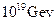Chapter 34, Problem 20PE

Chapter
Section
Textbook Problem

(a) Use the Heisenberg uncertainty principle to calculate the uncertainty in energy for a corresponding time interval of(b) Compare this energy with theunification−of−forces energy and discuss why they are similar.

To determine

(a)

The uncertainty in energy for the given time interval using Heisenberg uncertainty principle.

Explanation

Given:

Uncertainty in time interval, Δt=1043s

Plank's constant, h=6.62×1034J-s

Formula used:

Formula to calculate the uncertainty in energy for the given time interval using Heisenberg uncertainty principle is,

ΔE=h4πΔt ...... (I)

Where,

• ΔE is uncertainty in energy.
• h is Plank's constant.
• Δt is uncertainty in time interval.

Calculation:

Substitute 6.62×1034J-s for h and 1043s for Δt in equation (I).

ΔE=6

To determine

(b)

The relation between the uncertainty in energy and unification of force energy whether they are similar or not.

Still sussing out bartleby?

Check out a sample textbook solution.

See a sample solution

The Solution to Your Study Problems

Bartleby provides explanations to thousands of textbook problems written by our experts, many with advanced degrees!

Get Started

Calculate your protein RDA.

Understanding Nutrition (MindTap Course List)

Why are mathematical results rounded?

An Introduction to Physical Science

What biological evidence supports plate tectonics theory?

Oceanography: An Invitation To Marine Science, Loose-leaf Versin